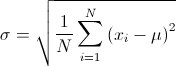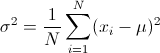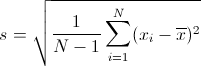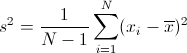×

# Standard Deviation Calculator

Enter numbers separated by comma, space or line break:

## About Standard Deviation Calculator

The online Standard Deviation Calculator is used to calculate the mean, variance, and standard deviation of a set of numbers.

## Standard Deviation

Standard deviation is a widely used measurement of variability or diversity used in statistics and probability theory. It shows how precise your data is.

The standard deviation is the square root of its variance. A low standard deviation indicates that the data points tend to be very close to the mean, whereas high standard deviation indicates that the data are spread out over a large range of values.

Variance and standard deviation depend on the mean of a set of numbers. Calculating them depends on whether the set is a population or sample.

## Variance and Standard Deviation of a Population

The population standard deviation is measures the variability of data in a population. It is usually an unknown constant. The variance σ2 and standard deviation σ of the population are given by:Where:
σ = population standard deviation
σ2 = population variance
x1, ..., xN = the population data set
μ = mean of the population data set
N = size of the population data set

## Variance and Standard Deviation of a Sample

The sample standard deviation is an estimate, based on a sample, of a population standard deviation. The variance s2 and standard deviation s of the sample are given by:Where:
s = sample standard deviation
s2 = sample variance
x1, ..., xN = the sample data set
x̄ = mean value of the sample data set
N = size of the sample data set

## Related

Watch Videos:

©2019 Miniwebtool | Terms and Disclaimer | Privacy Policy | Contact Us